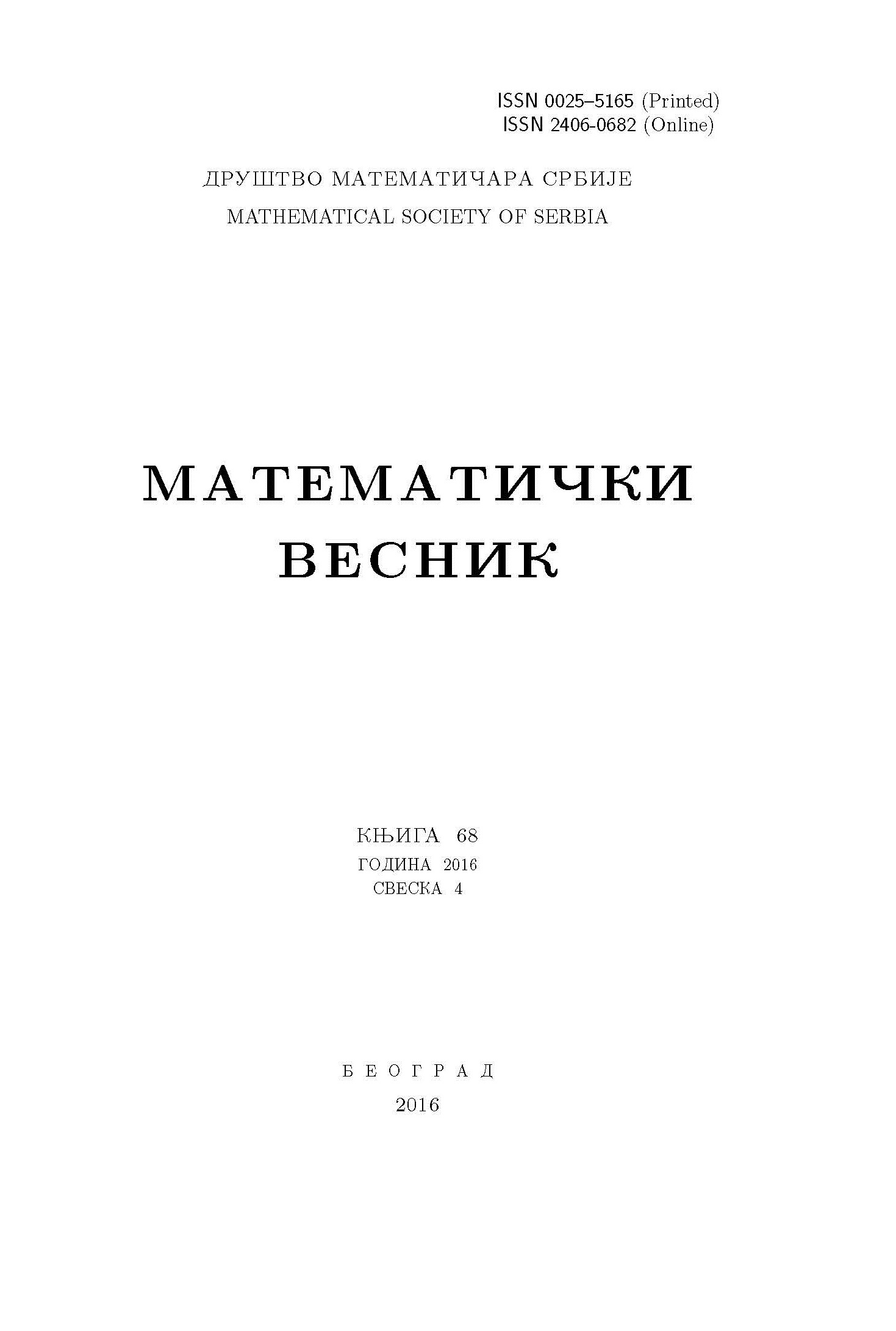﻿ Matematički Vesnik ﻿
 MATEMATIČKI VESNIK МАТЕМАТИЧКИ ВЕСНИКCONSTRUCTION OF THE TYPE 2 DEGENERATE POLY-EULER POLYNOMIALS AND NUMBERS W. A. Khan AbstractIn this paper, we introduce type 2 degenerate poly-Euler polynomials and numbers, briefly called degenerate poly-Euler polynomials and numbers, by using the modified degenerate polyexponential function and derive several properties on these polynomials and numbers. In the last section, we also consider the type 2 degenerate unipoly-Euler polynomials attached to an arithmetic function, by using the modified degenerate polyexponential function and investigate some identities of these polynomials. In particular, we give some new explicit expressions and identities of degenerate unipoly polynomials related to special numbers and polynomials.Keywords: Modified degenerate polyexponential functions; degenerate poly-Euler polynomials; degenerate unipoly functions; degenerate unipoly-Euler polynomials. MSC: 11B73, 11B83, 05A19 Pages:  1$-$10

﻿